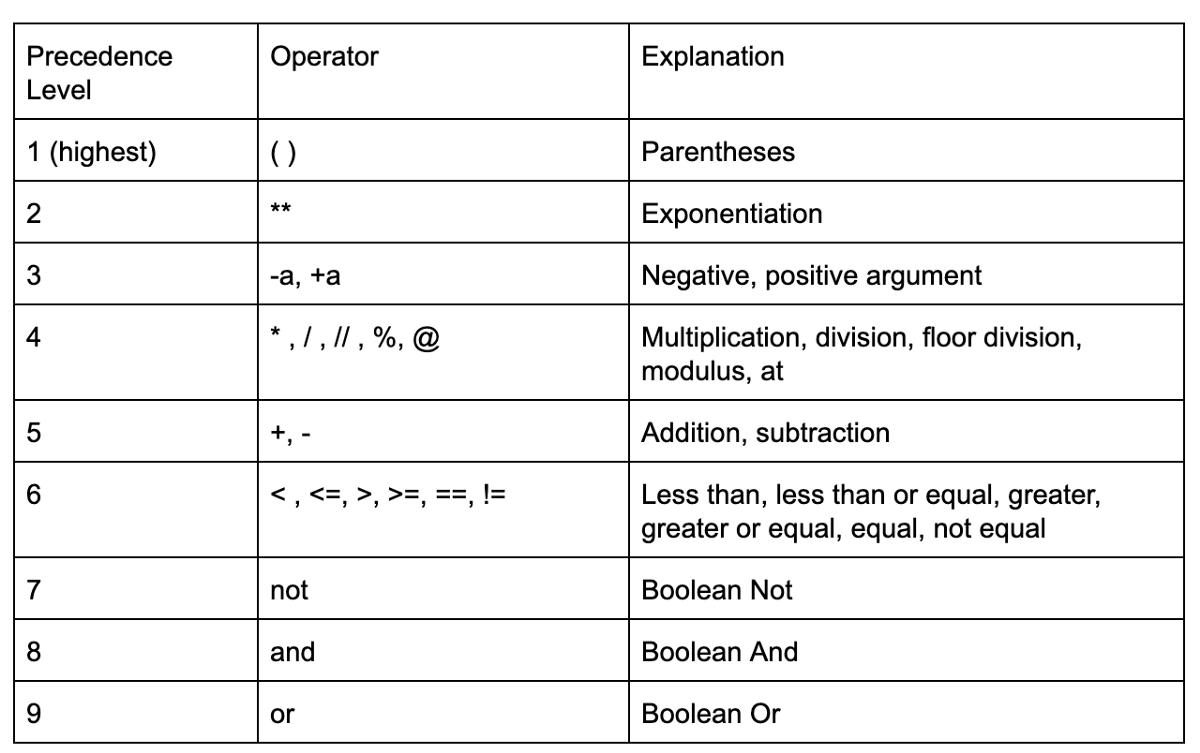# Order of Operations in Python

Manav Narula Jan 12, 2022

We have a variety of operators in Python, like arithmetical, logical, and more. An expression is a combination of operators, operands, variables. Sometimes, it isn’t easy to evaluate an expression due to many operators, and it is not clear which operator should be evaluated first.

For example, if we evaluate the `+` operator first in `10 + 2 / 2`, we get `12/2`, which is 6. However, if we evaluate `/` first, we get `10 + 1`, which is 11.

Therefore, the order in which operators will execute is important. In Python, we determine this using the Precedence of Operators. It determines which operators will execute first in an expression. Expressions in Python are usually executed from left to right.

The complete list of the order of operators from high to low is given below.It is simple to remember the above list using `PEMDAS`. Here, `P` means parentheses, `E` means exponential, `MD` means multiplication and division as both have the same precedence, and `AS` stands for addition and subtraction.

When operators have the same precedence, the one occurring first is executed.

Take the following example,

``````a = 10 * 5 + 2 / (8 + 2)
print(a)
``````

Output:

``````50.2
``````

Let us break down the above example. First, the expression within the parentheses, which comes out to be 10. Then the `*` operator is executed, calculating `10*5`, 50. The `/` operator calculates `2/10`, which is 0.2. Finally, the `+` operator evaluates `50 + 0.2`, which is 50.2.

We perform much more complicated calculations in Python with more complex expressions. The parentheses have the highest precedence, as shown in the previous example. Thus they are used in such complex expressions to group the sub-expressions accordingly to control which operators evaluate first.

Author: Manav Narula

Manav is a IT Professional who has a lot of experience as a core developer in many live projects. He is an avid learner who enjoys learning new things and sharing his findings whenever possible.